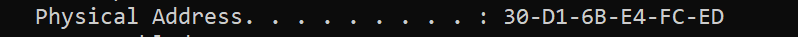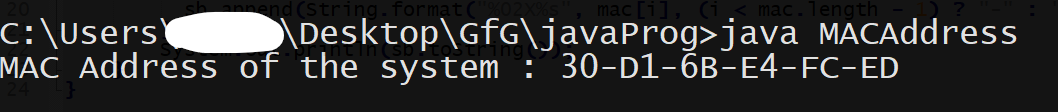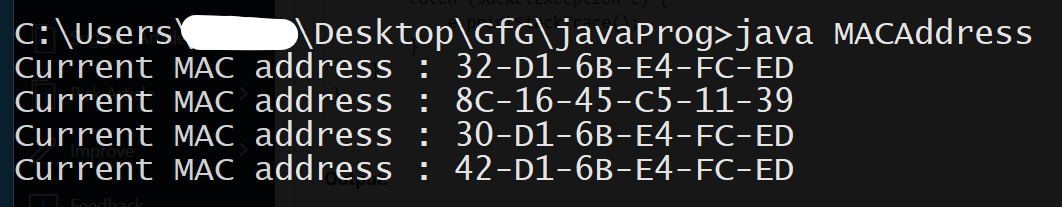# Java Program to Get System MAC Address of Windows and Linux Machine

• Difficulty Level : Easy
• Last Updated : 04 Jan, 2021

Media Access Control address (MAC address) is a unique hexadecimal identifier assigned to a Network Interface Controller (NIC) to be used as a network address in communications within a network segment. This use is common in most IEEE 802 networking technologies, including Ethernet, Wi-Fi, and Bluetooth. Within the Open Systems Interconnection (OSI) network model, MAC addresses utilized in the medium access control protocol sublayer of the data link layer. As typically represented, MAC addresses are recognizable as six groups of two hexadecimal digits, separated by hyphens, colons, or without a separator.Example 1

## Java

 `// Java program to access the MAC address of the ` `// localhost machine ` `import` `java.net.InetAddress; ` `import` `java.net.NetworkInterface; ` `import` `java.net.SocketException; ` `import` `java.net.UnknownHostException; ` `import` `java.util.Enumeration; ` `public` `class` `MACAddress { ` ` `  `    ``// method to get the MAC Address ` `    ``void` `getMAC(InetAddress addr) ``throws` `SocketException ` `    ``{ ` `        ``// create a variable of type NetworkInterface and ` `        ``// assign it with the value returned by the ` `        ``// getByInetAddress() method ` `        ``NetworkInterface iface ` `            ``= NetworkInterface.getByInetAddress(addr); ` ` `  `        ``// create a byte array and store the value returned ` `        ``// by the NetworkInterface.getHardwareAddress() ` `        ``// method ` `        ``byte``[] mac = iface.getHardwareAddress(); ` `       `  `        ``// convert the obtained byte array into a printable ` `        ``// String ` `        ``StringBuilder sb = ``new` `StringBuilder(); ` `        ``for` `(``int` `i = ``0``; i < mac.length; i++) { ` `            ``sb.append(String.format( ` `                ``"%02X%s"``, mac[i], ` `                ``(i < mac.length - ``1``) ? ``"-"` `: ``""``)); ` `        ``} ` `       `  `        ``// print the final String containing the MAC Address ` `        ``System.out.println(sb.toString()); ` `    ``} ` ` `  `    ``// Driver method ` `    ``public` `static` `void` `main(String[] args) ``throws` `Exception ` `    ``{ ` `        ``// a variable of type InetAddress to store the ` `        ``// address of the local host ` `        ``InetAddress addr = InetAddress.getLocalHost(); ` `       `  `        ``// instantiate the MACAddress class ` `        ``MACAddress obj = ``new` `MACAddress(); ` `        ``System.out.print(``"MAC Address of the system : "``); ` `       `  `        ``// call the getMAC() method on the current object ` `        ``// passing the localhost address as the parameter ` `        ``obj.getMAC(addr); ` `    ``} ` `}`

OutputExample 2(When the device has more than one MAC address)

## Java

 `// Java program to access all the MAC addresses of the ` `// localhost machine ` ` `  `import` `java.net.InetAddress; ` `import` `java.net.NetworkInterface; ` `import` `java.net.SocketException; ` `import` `java.net.UnknownHostException; ` `import` `java.util.Enumeration; ` `public` `class` `MACAddress { ` `    ``public` `static` `void` `main(String[] args) ``throws` `Exception ` `    ``{ ` `        ``// instantiate the MACAddress class ` `        ``MACAddress obj = ``new` `MACAddress(); ` `       `  `        ``// call the getMAC() method on the current object ` `        ``// passing the localhost address as the parameter ` `        ``obj.getMAC(); ` `    ``} ` ` `  `    ``// method to get the MAC addresses of the ` `    ``// localhost machine ` `    ``void` `getMAC() ` `    ``{ ` `        ``try` `{ ` `           `  `            ``// create an Enumeration of type ` `            ``// NetworkInterface and store the values ` `            ``// returned by ` `            ``// NetworkInterface.getNetworkInterfaces() ` `            ``// method ` `            ``Enumeration networks ` `                ``= NetworkInterface.getNetworkInterfaces(); ` `           `  `            ``// for every network in the networks Enumeration ` `            ``while` `(networks.hasMoreElements()) { ` `                ``NetworkInterface network ` `                    ``= networks.nextElement(); ` `               `  `                ``// call getHardwareAddress() method on each ` `                ``// network and store the returned value in a ` `                ``// byte array ` `                ``byte``[] mac = network.getHardwareAddress(); ` ` `  `                ``if` `(mac != ``null``) { ` `                    ``System.out.print( ` `                        ``"Current MAC address : "``); ` `                   `  `                    ``// convert the obtained byte array into ` `                    ``// a printable String ` `                    ``StringBuilder sb = ``new` `StringBuilder(); ` `                    ``for` `(``int` `i = ``0``; i < mac.length; i++) { ` `                        ``sb.append(String.format( ` `                            ``"%02X%s"``, mac[i], ` `                            ``(i < mac.length - ``1``) ? ``"-"` `                                                 ``: ``""``)); ` `                    ``} ` `                   `  `                    ``// print the final String containing the ` `                    ``// MAC Address ` `                    ``System.out.println(sb.toString()); ` `                ``} ` `            ``} ` `        ``} ` `        ``catch` `(SocketException e) { ` `            ``e.printStackTrace(); ` `        ``} ` `    ``} ` `}`

OutputMy Personal Notes arrow_drop_up
Recommended Articles
Page :